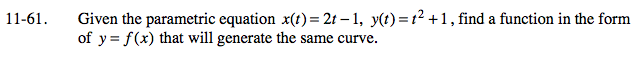Home > PC > Chapter 11 > Lesson 11.1.4 > Problem11-61

11-61.

Given the parametric equation x(t) = 2t − 1, y(t) = t2 + 1, find a function in the form of y = f (x) that will generate the same curve. Homework Help ✎Convert the x(t) equation to t = form.

Substitute your t = equation into the y(t) equation.